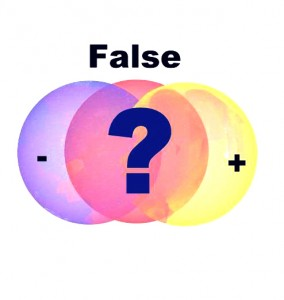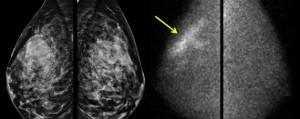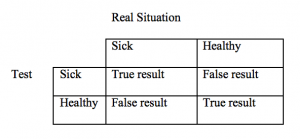# False Positive vs. False Negative

A guest post by Long Nguyen:

Not all results of medical tests are absolutely correct. Scientists can make mistakes when they conclude that something is true when it is actually false or that something is false when it is actually true.When something is concluded true and it is actually false, we have a false positive or type I error. On the other hand, when something is false and it is actually true, we have a false negative or type II error.

Should we really be worried about a positive medical test for a rare disease?

Mammography is one way to detect breast cancer at its early stage, which helps patients to increase their survivalrates. In 2009, John Allen Paulos, a mathematics professor at Temple University, wrote an article, Manmogram Math, to discuss how frequent women should have their mammograms. If mammograms could help diagnose breast cancer, why wouldn’t we have them more frequently? According to Paulos, it is controversial whether women should have mammograms monthly since the tests could cause harmful effects resulting from radiation. Also, suppose you have a positive test, is this absolutely true that you have cancer? The answer is no.

So how worried should you be if you have a positive test of a rare disease? In other words, how accurate is the test? Lisa Goldberg, a statistics professor at UC Berkeley, briefly explained how we should approach this problem on a Numberphile’s video, Are you REALLY sick? (false positives). Goldberg discussed some possible outcomes that the tests can bring to us, which is summarized in the table below:As we can see, there are two ways a test can fail to identify ‘real’ sick people. First, the test turns out negative while the test subject is really sick. In this case, it is a false negative. Second, the test can be positive while the test subject is really healthy, which is a false positive. Thus, it is important to measure the accuracy of the test when you receive positive test. In order to do that, we can find the probability of the sickness given a positive result, P(Sickness/Positive Result). Apply Bayes’ rule to this problem, we have:$P(\text{Sickness}\mid\text{Positive Result}) = \dfrac{P(\text{Positive Result}\mid\text{Sickness})\cdot P(\text{Sickness})}{P(\text{Positive Result})}$

and\begin{aligned}P(\text{Positive Result}) = &P(\text{Positive Result}\mid\text{Sickness})\cdot P(\text{Sickness})\\ &+ P(\text{Positive Result}\mid \text{Healthy})\cdot P(\text{Healthy})\end{aligned}

Suppose we have a rare disease which happens in 1 out of 1000 people; the test is 99% accurate to tell real sick people and the test misdiagnoses 1 out of 10 healthy people as sick. According to our assumption,$P(\text{Positive Result}\mid \text{Sickness}) = 0.99;$$P(\text{Sickness}) = \text{1}{1000} = 0.001;$$P(\text{Positive Result}\mid\text{Healthy}) = \dfrac{1}{10} = 0.1;$$P(\text{Healthy}) = 1-\dfrac{1}{1000} = 0.999.$

As a result,$P(\text{Positive Result}) = (0.99)(0.001) + (0.1)(0.999) \approx 0.1$

Thus,$P(\text{Sickness}\mid\text{Positive Result}) = \dfrac{(0.99)(0.001)}{0.1} \approx 0.01.$

Given the rare disease and a test that can mostly identify all sick people and give 1 false positive in every 10 test subjects, your chance of having the disease is 0.01, or 1 out of 100. So it is always a good idea to consult second opinion.All medical tests can be resulted in false positive and false negative errors. Since medical tests can’t be absolutely true, false positive and false negative are two problems we have to deal with. A false positive can lead to unnecessary treatment and a false negative can lead to a false diagnostic, which is very serious since a disease has been ignored. However, we can minimize the errors by collecting more information, considering other variables, adjusting the sensitivity (true positive rate) and specificity (true negative rate) of the test, or conducting the test multiple times. Even so, it is still hard since reducing one type of error means increasing the other type of error. Sometimes, one type of error is more preferable than the other one, so scientists will have to evaluate the consequences of the errors and make a decision.

References:
 Paulos, J. A. (2009, December 10). Mammogram Math [Web]. Retrieved from http://www.nytimes.com/2009/12/13/magazine/13Fob-wwln-t.html?_r=2
 Haran, B. [Numberphile]. (2016, March 16). Are you REALLY sick? (false positives) [Video file]. Retrieved from https://www.youtube.com/watch?v=M8xlOm2wPAA
 Fernandez, E. (2011, October 17). High Rate of False-Positives with Annual Mammograms [Web]. Retrieved from https://www.ucsf.edu/news/2011/10/10778/high-rate-false-positives-annual-mammogram
Images:
https://si.wsj.net/public/resources/images/BN-HB837_HJourn_F_20150223124818.jpg1.NOVIAN says: